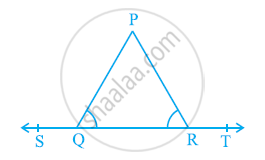# In the given figure, ∠PQR = ∠PRQ, then prove that ∠PQS = ∠PRT - Mathematics

In the given figure, ∠PQR = ∠PRQ, then prove that ∠PQS = ∠PRT.#### Solution

In the given figure, ST is a straight line and ray QP stands on it.

∴ ∠PQS + ∠PQR = 180º (Linear Pair)

∠PQR = 180º − ∠PQS .........(1)

∠PRT + ∠PRQ = 180º (Linear Pair)

∠PRQ = 180º − ∠PRT ...........(2)

It is given that ∠PQR = ∠PRQ.

Equating equations (1) and (2), we obtain

180º − ∠PQS = 180° − ∠PRT

∠PQS = ∠PRT

Concept: Pairs of Angles
Is there an error in this question or solution?
Chapter 6: Lines and Angles - Exercise 6.1 [Page 97]

#### APPEARS IN

NCERT Class 9 Maths
Chapter 6 Lines and Angles
Exercise 6.1 | Q 3 | Page 97

Share